Zonal harmonics

zonal harmonic polynomials

Zonal harmic polynomials are spherical harmonic polynomials (cf. also Spherical harmonics) that assume constant values on circles centred on an axis of symmetry. They characterize single-valued harmonic functions on simply-connected domains with rotational symmetry.

To be specific, one introduces the spherical coordinates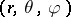as,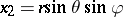,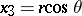, where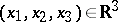. The zonal harmonics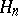are the polynomial solutions of the Laplace equationthat are axially symmetric (i.e. independent of the angle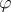). They can be expressed in terms of Legendre polynomials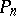of degree, as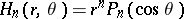for, and form a complete orthogonal set of functions in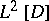, where: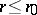. Thevanish on cones that divide a sphere centred at the origin intozones, hence the name zonal harmonics. Theare sometimes referred to as solid zonal harmonics and theas surface zonal harmonics.

Applications.

Two types of applications arise in classical potential theory (see [a4], [a6], [a7]).

In the first, one determines the potential in a sphere from its boundary values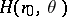. By specifying appropriate regularity conditions, the orthogonality of the Legendre polynomials is used to expandas the Fourier–Legendre series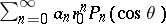. The potential in the sphere is recovered as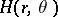. The exterior boundary value problem is formulated by means of the Kelvin transformation. The potential between two concentric spheres is determined by combining solutions of the interior and the exterior problems.

In the second, one determines the potential at points in space from its values on a segment of the symmetry axis. The solution relies on the fact that along this axis the zonal harmonics,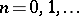. Thus, if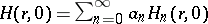, then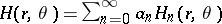for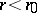, where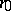is the radius of convergence of the Taylor series.

Relation with analytic functions.

There are many connections between the properties of the potentialsand those of analytic functionsof a complex variable (cf. also Analytic function; Harmonic function). One such connection, related to the previous example, concerns singularities and uses the generating function for zonal harmonics to construct reciprocal integral transforms connectingwith. The following fact is immediate (see [a3], [a8]). Let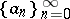be a sequence of real constants for which. Consider the associated harmonic and analytic functionsand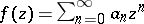, which are regular for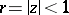. Then the boundary point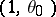is a singularity ofif and only if the boundary pointis a singularity of. Thus, the singularities of solutions of a singular partial differential equation are characterized in terms of those of associated analytic functions and vice versa.

From the 1950s onwards, an extensive literature has developed using integral transform methods to study solutions of large classes of multi-variable partial differential equations. The analysis is based on the theory of analytic and harmonic functions in several variables. Zonal harmonics play an important role in axially symmetric problems in(see [a1], [a2], [a3], [a5]).